Most Affordable JEE | NEET | 8,9,10 Preparation by Kota's Top IITian Doctor Faculties

# Caboxylic Acid - JEE Advanced Previous Year Questions with SolutionsJEE Advanced Previous Year Questions of Chemistry with Solutions are available at eSaral. Practicing JEE Advanced Previous Year Papers Questions of Chemistry will help the JEE aspirants in realizing the question pattern as well as help in analyzing weak & strong areas. Simulator Previous Years JEE Advance Questions
Q. Match each of the compound in Column I with its characteristic reaction(s) in Column II.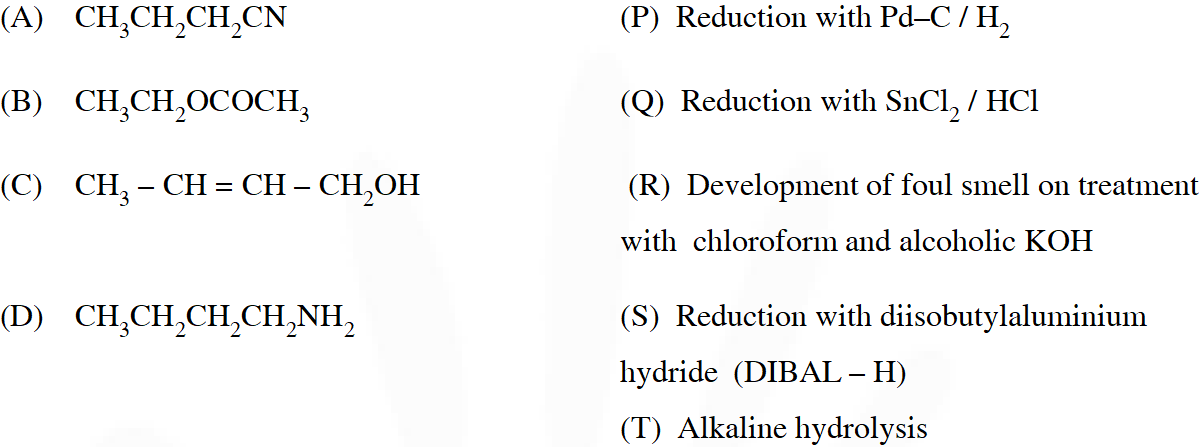[IIT 2009]
Ans.
Q. Match each of the compounds given in Column I with the reaction(s), that they can undergo, given in Column II.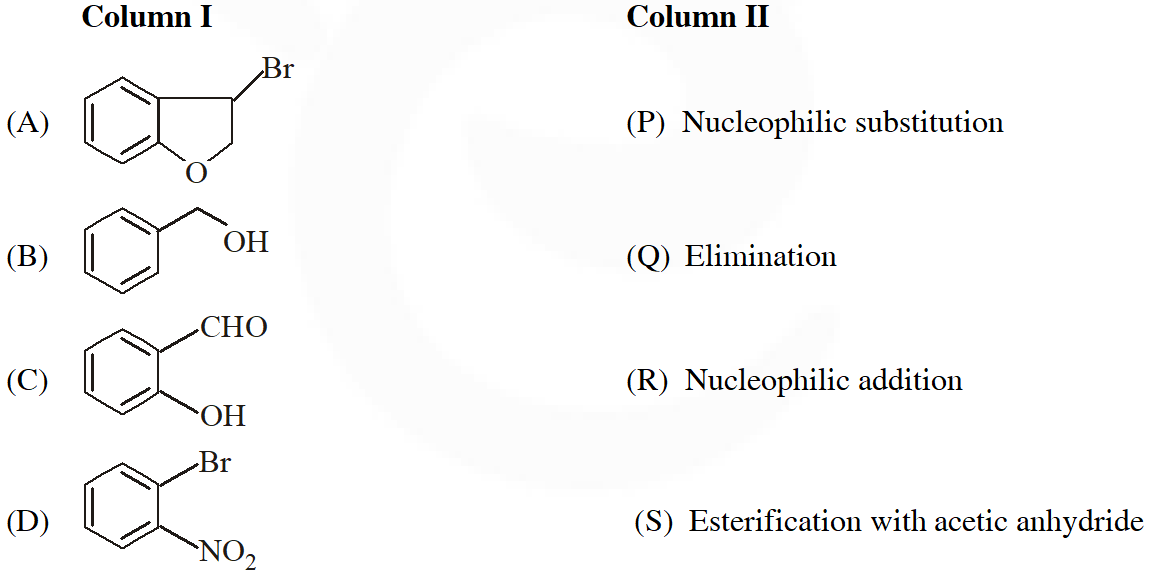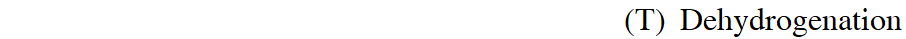[IIT 2009]
Ans. ($(A) \rightarrow P, Q, S, T:(B) \rightarrow P, S, T ;(C) \rightarrow P:(D) \rightarrow R$)
Q. The major product of the following reaction is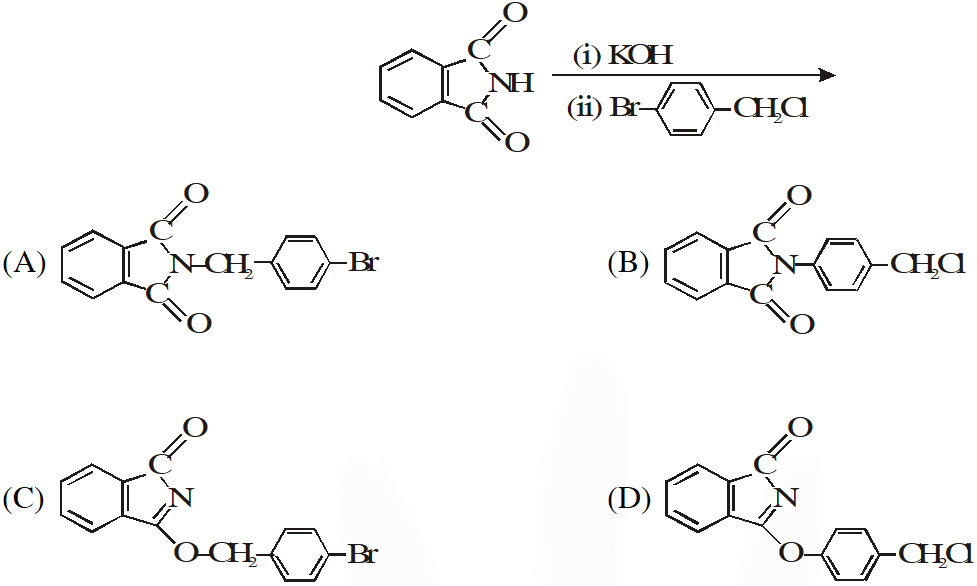[IIT 2011]
Ans. ($(\mathrm{A}) \rightarrow \mathrm{P}, \mathrm{Q}, \mathrm{T} ;(\mathrm{B}) \rightarrow \mathrm{P}, \mathrm{Q}, \mathrm{S}, \mathrm{T},(\mathrm{C}) \rightarrow \mathrm{R}, \mathrm{S}, ;(\mathrm{D}) \rightarrow \mathrm{P}$)
Q. With reference the scheme given, which of the given statement(s) about T, U, V & W is (are) correct ?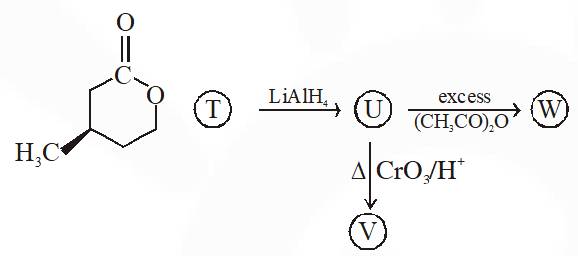(A) 'T' is soluble in hot aq NaOH (B) 'U' is optically active (C) mol formula of $\mathrm{W}$ is $\mathrm{C}_{10} \mathrm{H}_{18} \mathrm{O}_{4}$ (D) V gives effervescence with aq NaHCO $_{3}$ [IIT 2012]
Ans. (A)
Q. Identify the binary mixtures (s) that can be separated into the individual compounds, by differential extraction, as shown in the given scheme –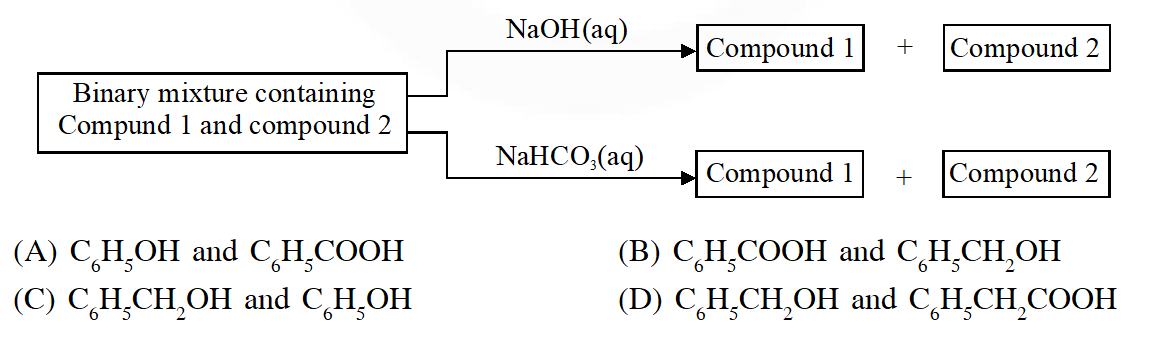[IIT 2012]
Ans. (A,C,D)
Q. The total number of carboxylic acid groups in the product P is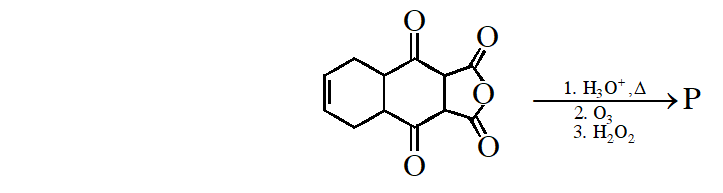[IIT 2013]
Ans. (B,D)
Q. In the reaction shown below, the major product(s) formed is / are :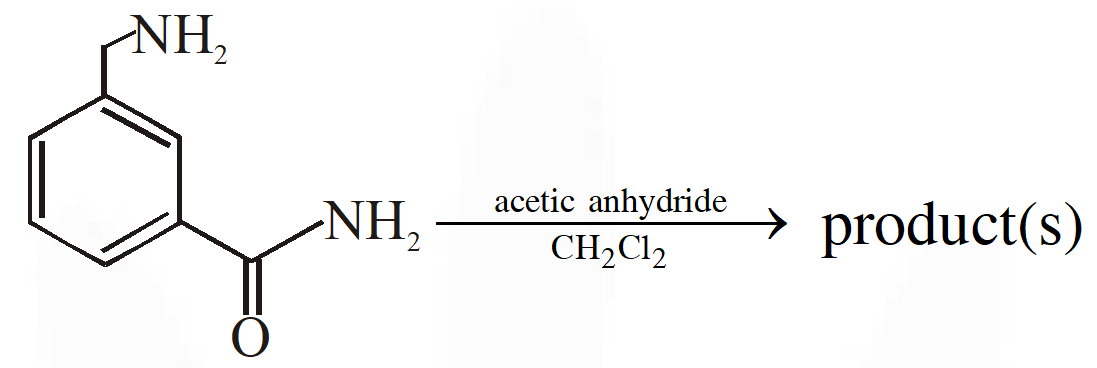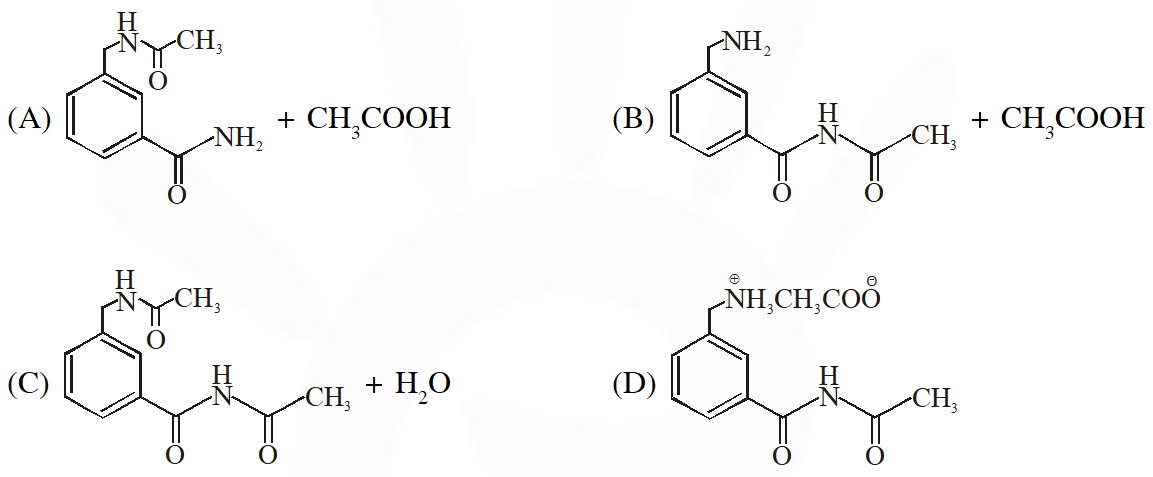[IIT 2014]
Ans. 2
Q. Different possible thermal decomposition pathways for peroxyesters are shown below. Match each pathway from List-I with an appropriate structure from List-II and select the correct answer using the code given below the lists.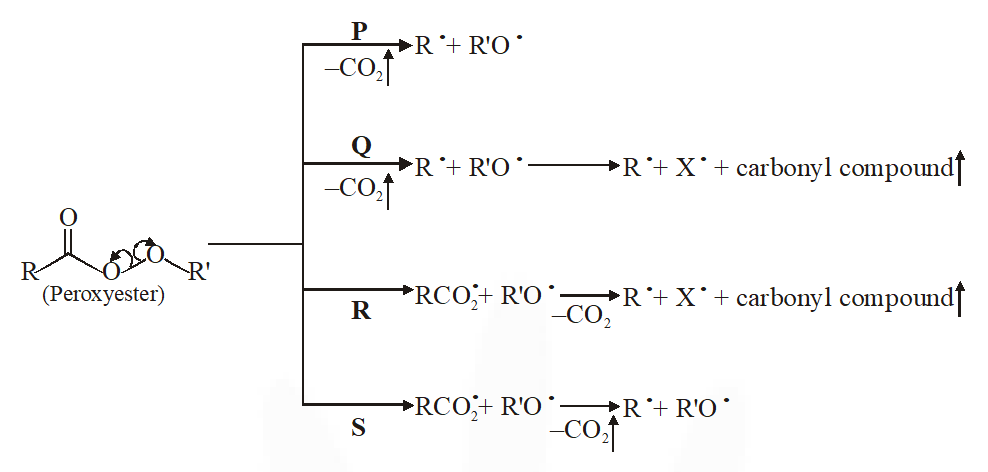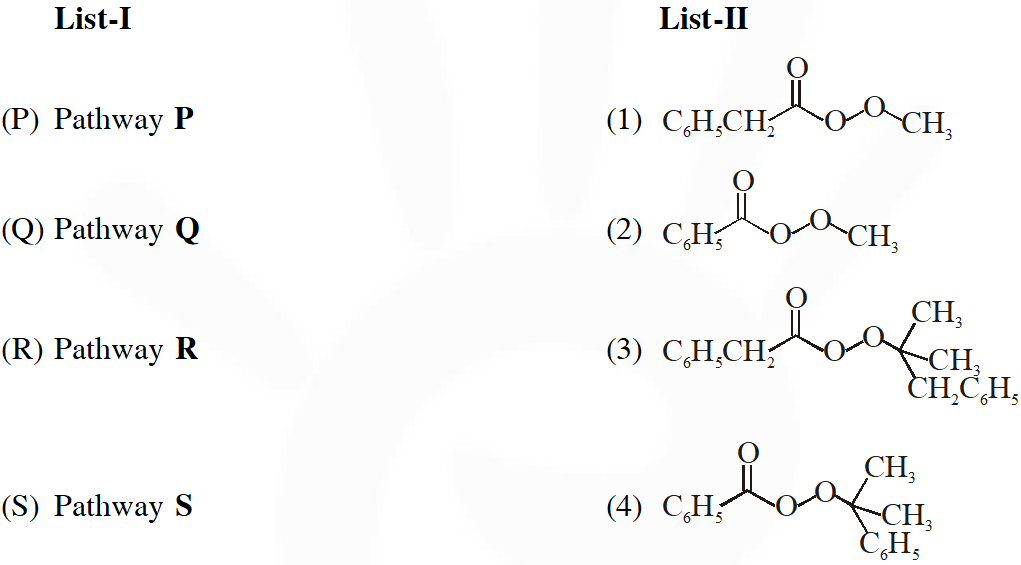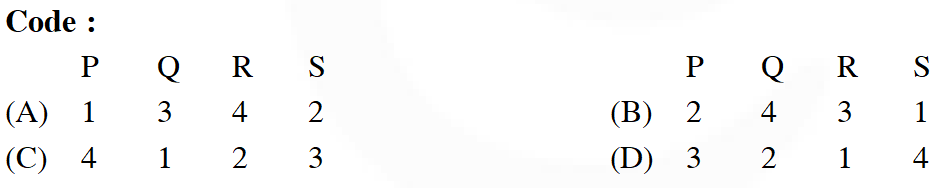[IIT 2014]
Ans. (A)
Q. The major product of the reaction is :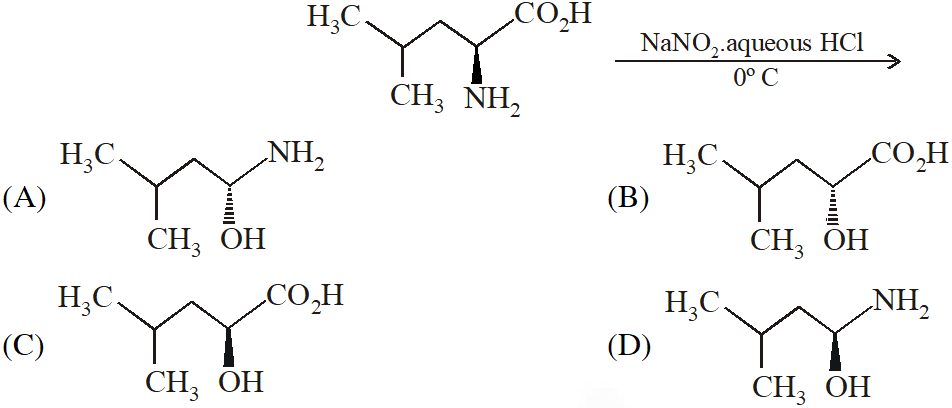[IIT 2015]
Ans. (A)
Q. Aniline reacts with mixed acid (conc. HNO, and conc. $\mathrm{H}_{2} \mathrm{SO}_{4}$ ) at 288 K to give P (51%), Q (47%) and R (2%). The major product(s) the following reaction sequence is (are) :-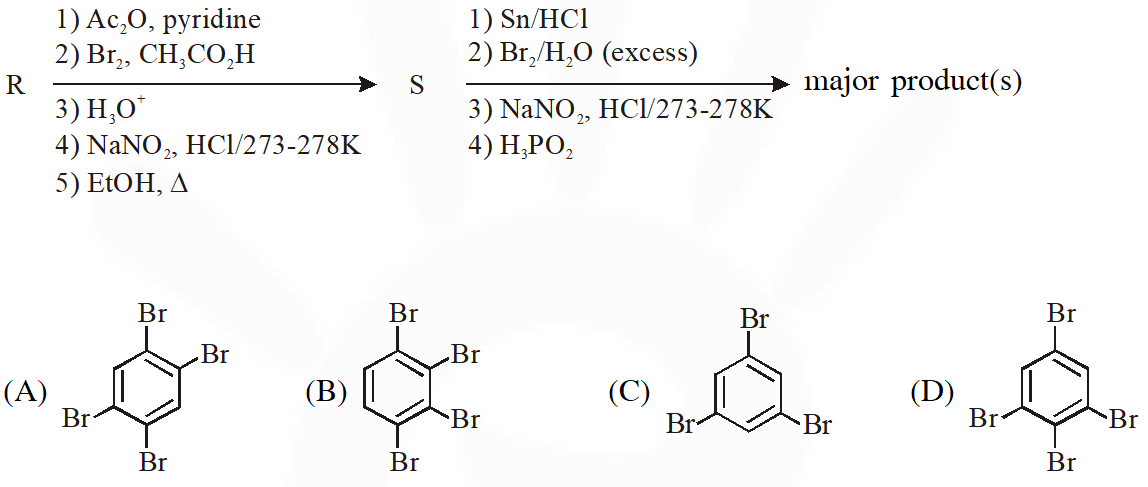[JEE Adv. 2018]
Ans. (C)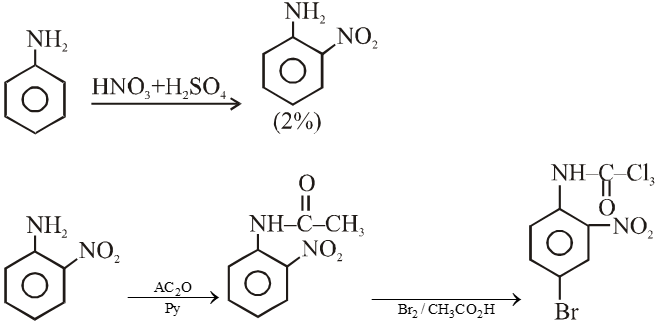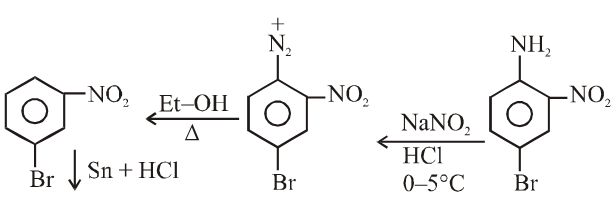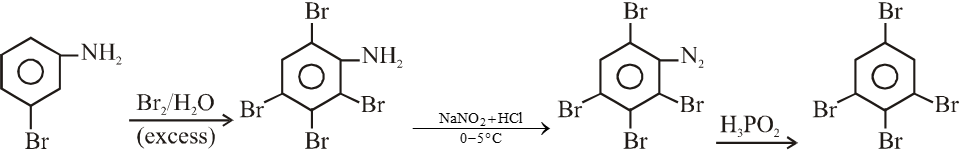Q. In the following reaction sequence, the correct structure(s) of X is (are)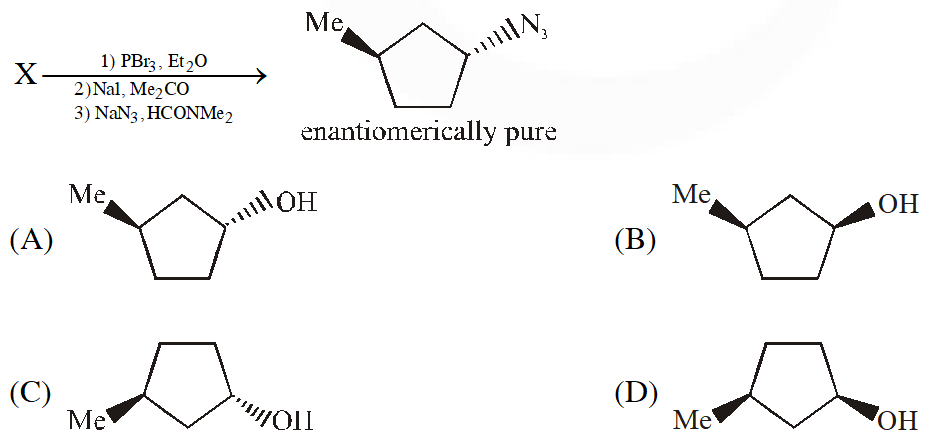[JEE Adv. 2018]
Ans. (D)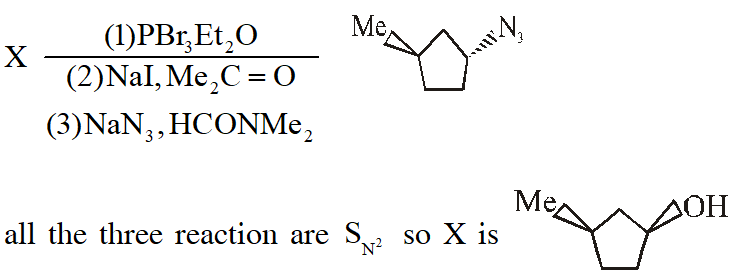Treatment of benzene with CO/HCl in the presence of anhydrous $\mathrm{AlCl}_{3} / \mathrm{CuCl}$ followed by reaction with Ac2O/NaOAc gives compound X as the major product. Compound X upon reaction with $\mathrm{Br}_{2} / \mathrm{Na}_{2} \mathrm{CO}_{3}$, followed by heating at 473 K with moist KOH furnishes Y as the major product. Reaction of X with $\mathrm{H}_{2} / \mathrm{Pd}-\mathrm{C}$, followed by $\mathrm{H}_{3} \mathrm{PO}_{4}$ treatment gives Z as the major product. (There are two questions based on PARAGRAPH "X", the question given below is one of them)
Q. The compound Y is :-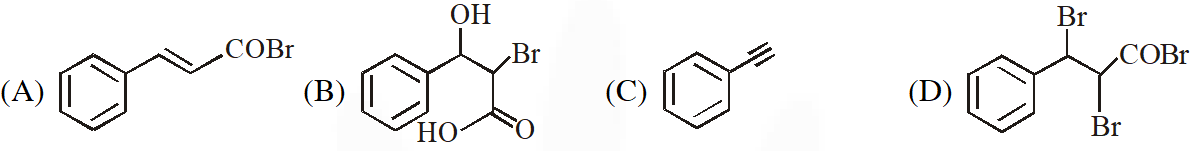[JEE Adv. 2018]
Ans. (B)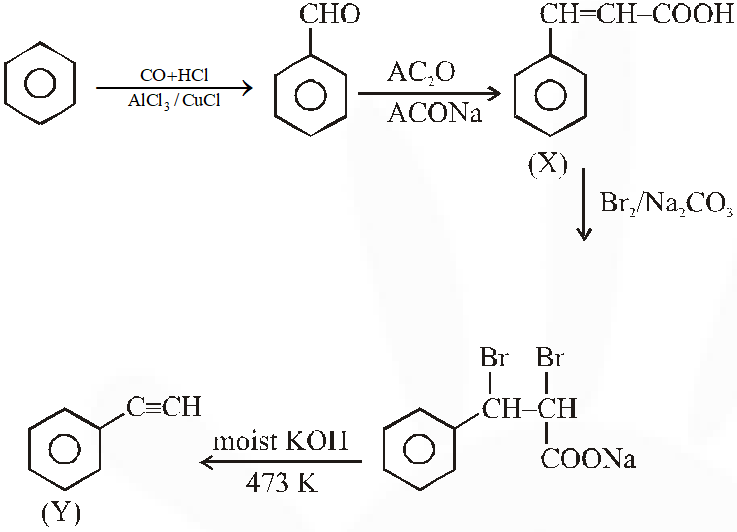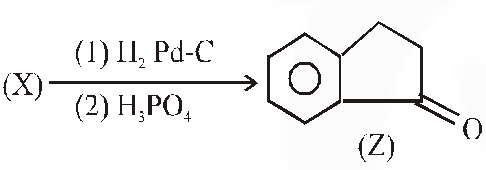Q. The compound Z is :-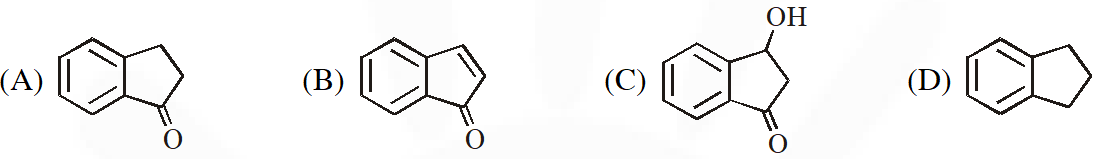[JEE Adv. 2018]
Ans. (C)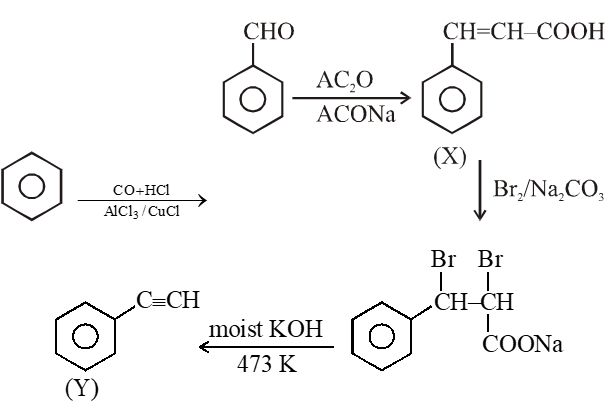An organic acid P $\left(\mathrm{C}_{1} \mathrm{H}_{12} \mathrm{O}_{2}\right)$ can easily be oxidized to a dibasic acid which reacts with ethyleneglycol to produce a polymer dacron. Upon ozonolysis, P gives an aliphatic ketone as one of the products. P undergoes the following reaction sequences to furnish R via Q. The compound P also undergoes another set of reactions to produce S.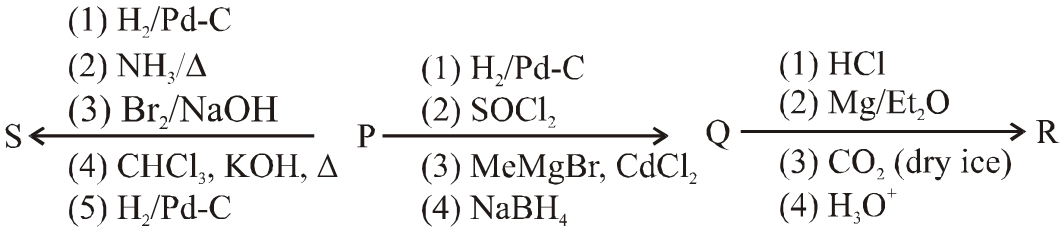(There are two questions based on PARAGRAPH "A", the question given below is one of them)
Q. The compound R is (A)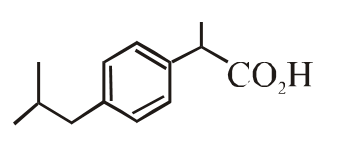(B)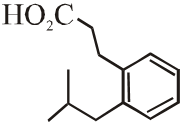(C)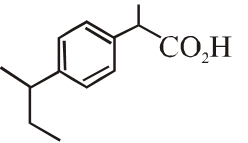(D)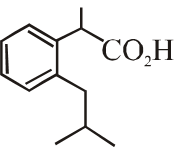[JEE Adv. 2018]
Q. The compound S is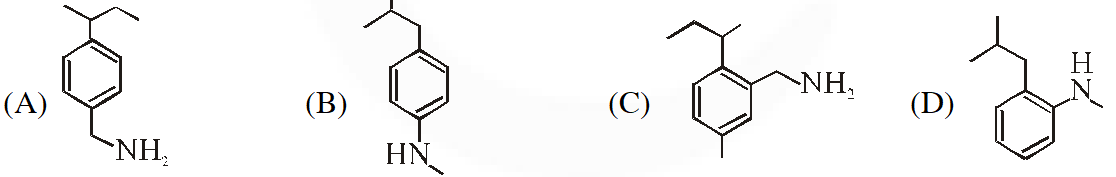[JEE Adv. 2018]